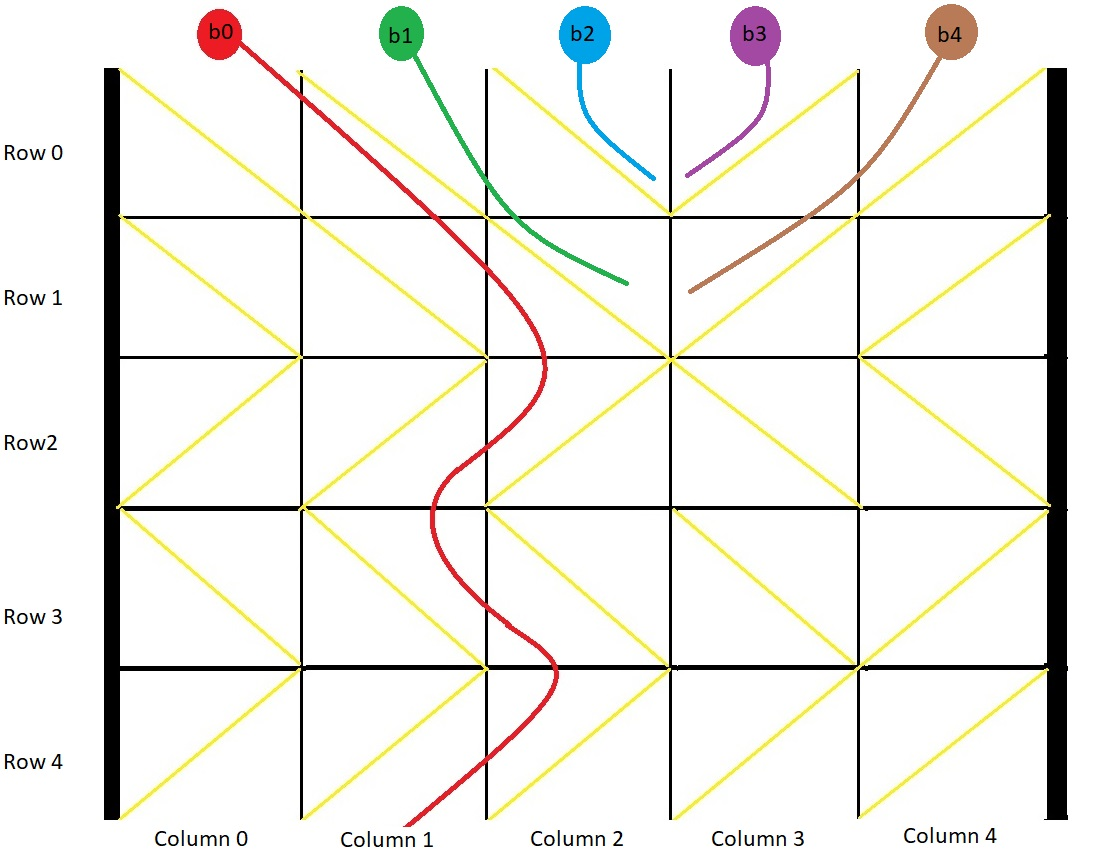1706. Where Will the Ball Fall

You have a 2-D `grid` of size `m x n` representing a box, and you have `n` balls. The box is open on the top and bottom sides.

Each cell in the box has a diagonal board spanning two corners of the cell that can redirect a ball to the right or to the left.

• A board that redirects the ball to the right spans the top-left corner to the bottom-right corner and is represented in the grid as `1`.
• A board that redirects the ball to the left spans the top-right corner to the bottom-left corner and is represented in the grid as `-1`.

We drop one ball at the top of each column of the box. Each ball can get stuck in the box or fall out of the bottom. A ball gets stuck if it hits a "V" shaped pattern between two boards or if a board redirects the ball into either wall of the box.

Return an array `answer` of size `n` where `answer[i]` is the column that the ball falls out of at the bottom after dropping the ball from the `ith` column at the top, or `-1` if the ball gets stuck in the box.

Example 1:```Input: grid = [[1,1,1,-1,-1],[1,1,1,-1,-1],[-1,-1,-1,1,1],[1,1,1,1,-1],[-1,-1,-1,-1,-1]]
Output: [1,-1,-1,-1,-1]
Explanation: This example is shown in the photo.
Ball b0 is dropped at column 0 and falls out of the box at column 1.
Ball b1 is dropped at column 1 and will get stuck in the box between column 2 and 3 and row 1.
Ball b2 is dropped at column 2 and will get stuck on the box between column 2 and 3 and row 0.
Ball b3 is dropped at column 3 and will get stuck on the box between column 2 and 3 and row 0.
Ball b4 is dropped at column 4 and will get stuck on the box between column 2 and 3 and row 1.
```

Example 2:

```Input: grid = [[-1]]
Output: [-1]
Explanation: The ball gets stuck against the left wall.
```

Constraints:

• `m == grid.length`
• `n == grid[i].length`
• `1 <= m, n <= 100`
• `grid[i][j]` is `1` or `-1`.

1706. Where Will the Ball Fall
``````struct Solution;

impl Solution {
fn find_ball(grid: Vec<Vec<i32>>) -> Vec<i32> {
let n = grid.len();
let m = grid.len();
let mut res = vec![];
for j in 0..m {
res.push(Self::dp(0, j, &grid, n, m));
}
res
}

fn dp(i: usize, j: usize, grid: &[Vec<i32>], n: usize, m: usize) -> i32 {
if i == n {
j as i32
} else {
if grid[i][j] == 1 && j + 1 < m && grid[i][j + 1] == 1 {
return Self::dp(i + 1, j + 1, grid, n, m);
}
if grid[i][j] == -1 && j >= 1 && grid[i][j - 1] == -1 {
return Self::dp(i + 1, j - 1, grid, n, m);
}
-1
}
}
}

#[test]
fn test() {
let grid = vec_vec_i32![
[1, 1, 1, -1, -1],
[1, 1, 1, -1, -1],
[-1, -1, -1, 1, 1],
[1, 1, 1, 1, -1],
[-1, -1, -1, -1, -1]
];
let res = vec![1, -1, -1, -1, -1];
assert_eq!(Solution::find_ball(grid), res);
}
``````